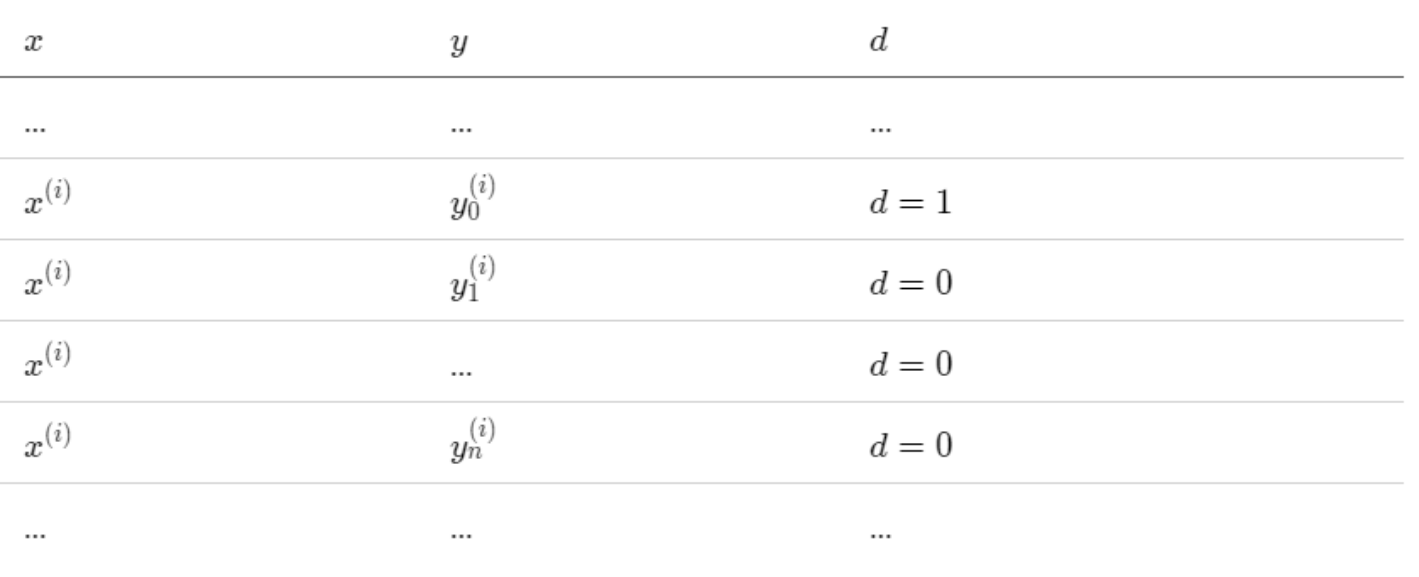# 【番外】负采样原理

## NCE（噪声对比估计）

$P(y=k|x) = \frac{\exp(w_k^T x + b_k)}{\sum_j exp(w_j^T x + b_j)} \\ \, \\ = \frac{\exp(w_k^T x + b_k)}{Z}$

NCE 的想法很简洁，把多分类变成二分类，还用相同的参数。$P(y | x, d = 1) = P_0(y | x)$

$P(y | x, d = 0) = N(y)$

$P(d = 1 | x) = \frac{1}{n + 1} \\ \, \\ P(d = 0 | x) = \frac{n}{x + 1}$

$P(d = 1, y | x) = \frac{1}{n + 1} P_0(y | x) \\ \, \\ P(d = 0, y | x) = \frac{n}{n + 1} N(y)$

$P(d = 1| y, x) = \frac{P(d = 1, y | x)}{P(d = 1, y | x) + P(d = 0, y | x)} \\ \, \\ = \frac{P_0(y | x)}{P_0(y | x) + nN(y)}$

## 负采样

$P(d = 1 | y, x) = \frac{P_0(y | x)}{P_0(y | x) + 1} \\ \, \\ = \frac{\exp(w_k^T x + b_k)}{\exp(w_k^T x + b_k) + z} \\ \, \\ = \frac{1}{1 + \exp(- w_k^T x - b_k + \log z)} \\ \, \\ = \sigma(w_k^T x + b_k - \log z)$

$P(d = 1 | y, x) = \sigma(w_k^T x + b_k)$

## 参考

07-281693

#### NLP 之 word2vec 以及负采样原理详解

05-253906

#### 推荐系统之---正负样本构造trick04-13962

#### 带权采样——word2vec负采样中的原理

05-25471

#### 深度总结 | 知识蒸馏在推荐系统中的应用

02-218644

#### 负采样的理解

03-021835

#### 负采样

12-19193

#### 通俗易懂的word2Vec负采样理解

07-051322

#### 负采样方法

12-062661

#### 深度学习NLP笔记（二）：Negative sampling（负采样）和Hierarchical softmax（层次softmax）

04-042700

#### （三）通俗易懂理解——Skip-gram的负采样

04-23423

#### Negative Sampling 负采样详解

08-242万+

#### Word2Vec导学第二部分 - 负采样

11-12361

#### 负采样（negative sampling））

12-085708

#### word_embedding的负采样算法,Negative Sampling 模型

04-281562

#### 负采样算法

03-153375

#### negative sampling负采样和nce loss

08-291735

#### word2vec 负采样思路

08-291387

#### [DeeplearningAI笔记]序列模型2.7负采样Negative sampling

03-221万+

#### word2vec的负采样算法

06-21244

#### Word2Vec Tutorial（2）- Negative Sampling 负采样（Stanford cs224n 词向量学习之拓展阅读2）©️2020 CSDN 皮肤主题: 黑客帝国 设计师: 上身试试点击重新获取扫码支付1.余额是钱包充值的虚拟货币，按照1:1的比例进行支付金额的抵扣。
2.余额无法直接购买下载，可以购买VIP、C币套餐、付费专栏及课程。余额充值